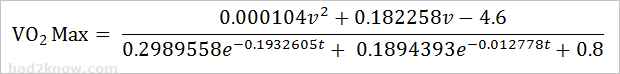# Race Results VO2 Max Calculator

## Daniels and Gilbert Formula for VO2 Max

VO2 Max Calculator (Race Results)
Distance Run meters
Running Time minutes
seconds

To convert distances in miles into meters,
use the conversion calculator.

VO₂ max is the maximal oxygen consumption or maximum aerobic capacity of an individual, it is the number of liters of oxygen one's body can transport per minute during incremental exercise (exercise that increase in intensity over time.) VO₂ max is a measure of physical fitness since people who are in better shape can use and transport more oxygen throughout their bodies during intense exercise. Besides L/min, VO₂ max may also be measured in milliliters of oxygen per kilogram of body weight per minute, or mL/kg/min.

A person's true VO₂ max can be measured in a clinical setting using treadmill and a device that records the O₂ and CO₂ concentrations in the air that is inhaled and exhaled. The maximal level is achieved when the body's consumption of oxygen holds steady even as the intensity of the exercise increases.

Since most people can't determine their VO₂ max level in an exercise lab, there are several approximation formulas. The Daniels and Gilbert formula estimates a runner's VO₂ max from the results of a race where the distance is at least 1500 meters (0.932 miles). This formula is programmed into the calculator and yields your level in mL/kg/min.

### The Daniels and Gilbert Equation for VO2 Max

Daniels and Gilbert devised an equation for approximating VO₂ max based on tabulated race results data. The equation is fairly complicated, but nonetheless widely used in the running world.In the equation, v = velocity in meters per minute, and t = time in minutes.

Example: Sara runs a 5 km race in 22 minutes and 50 seconds. Her time is 22.8333 minutes and her velocity is 218.9781 meters per minute. Plugging the values of v = 218.9781 and t = 22.833 into the Daniels and Gilbert VO₂ max formula gives

VO₂ Max = 40.29746/0.94512 = 42.64 mL/kg/min.